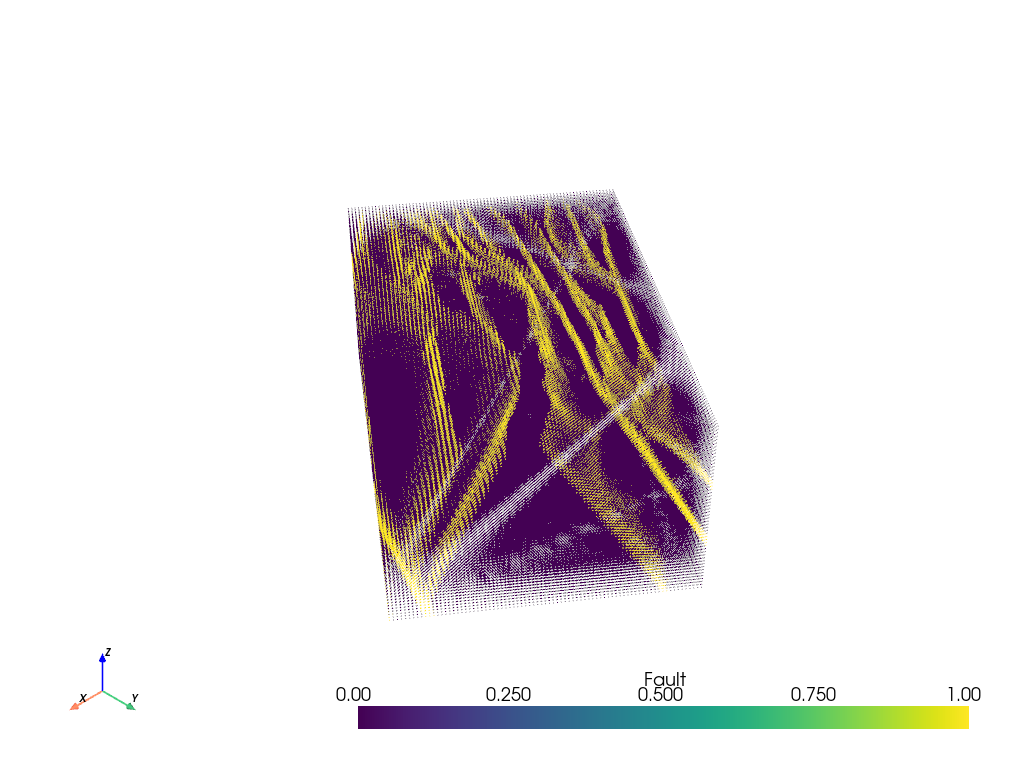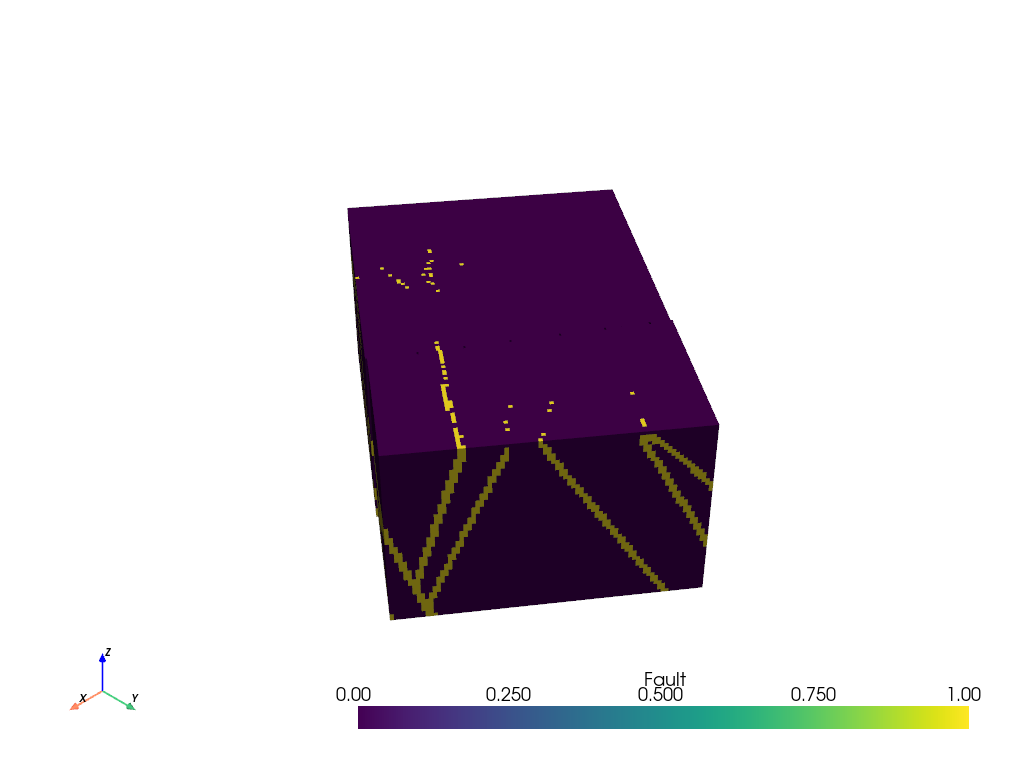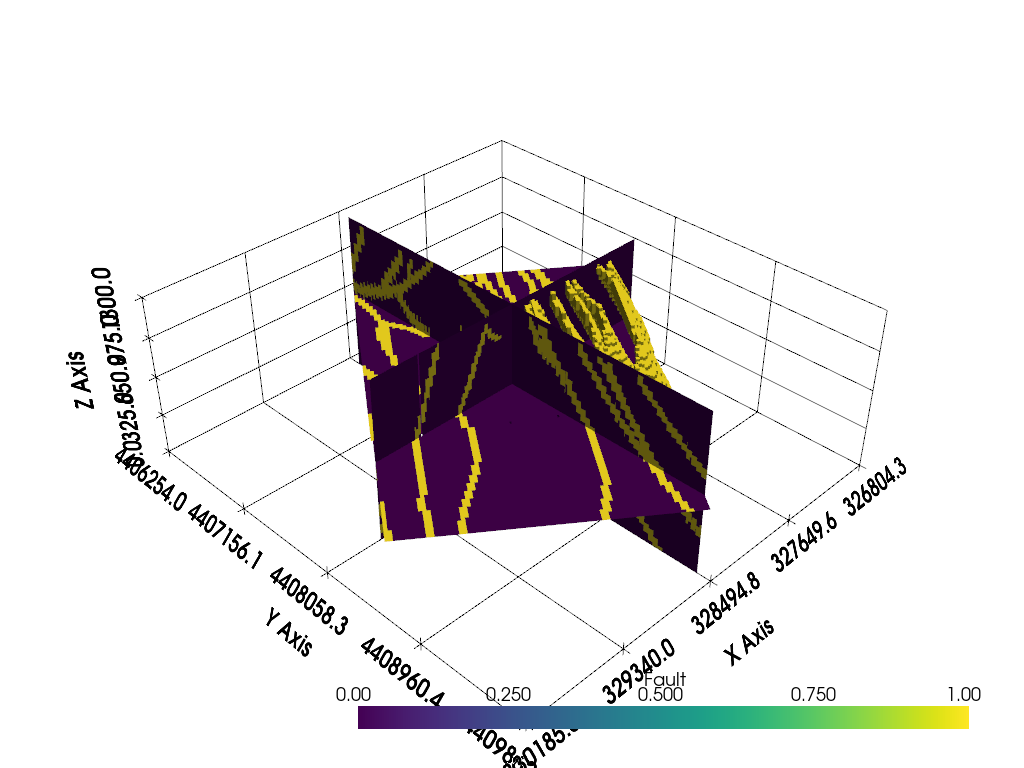# Voxelize Points¶

This example will demonstrate how to connect a set of points defined on a regular grid to create a vtkUnstructuredGrid which can be used to perform volumetric operations.

This example demos `PVGeo.filters.VoxelizePoints`

```# sphinx_gallery_thumbnail_number = 2
import pyvista
from pyvista import examples
import numpy as np
import pandas as pd
import PVGeo
from PVGeo.filters import VoxelizePoints
```

Download sample data files and keep track of names:

```url = 'https://github.com/OpenGeoVis/PVGeo/raw/master/tests/data/fault_points.csv'
fault_file, _ = examples.downloads._retrieve_file(url, 'fault_points.csv')
```

Let’s go ahead and load a simple file that has XYZ coordinates and a boolean array for fault presence. This point cloud makes some sort of regular grid, but we have forgotten the deatials of the cell spacings and local coordinate rotations.

We will read in this data with `pandas` and send it to the `PVGeo.points_to_poly_data()` helper to create a `pyvista.PolyData` object (essentially a point cloud).

```points = pd.read_csv(fault_file)
print(points[0:2])
```

Out:

```            X            Y       Z  Fault
0  326819.497  4407450.636  1287.5      0
1  326834.340  4407470.753  1287.5      0
```
```vtkpoints = PVGeo.points_to_poly_data(points)
print(vtkpoints)
```

Out:

```PolyData (0x7f9f05dd01c0)
N Cells:      499200
N Points:     499200
X Bounds:     3.268e+05, 3.302e+05
Y Bounds:     4.406e+06, 4.410e+06
Z Bounds:     1.250e+01, 1.288e+03
N Arrays:     1
```

Note that we have a `pyvista.PolyData` object now which allows us to do all types of immediate plotting of our data. First, lets threshold our points as the point cloud has a bunch of zeros and ones throughout the dataspace to describe the presence of a fault.

To threshold the points, we call the threshold filter directly on our data object and pass the thresholding value. We can then plot the result by calling the plot function. (Note: change the notebook parameter to `False` for an interactive window)

```vtkpoints.plot(clim=[0,1], point_size=1)
```Out:

```[(334141.1509516418, 4413705.151451642, 6293.877451641769),
(328497.2735, 4408061.274, 650.0),
(0.0, 0.0, 1.0)]
```

## Points to Voxelized Volume¶

The above figure is pretty cool! But its a point cloud which means out filtering options are pretty limited. Fortunately, we know that the point cloud represents some sort of regularized gridded volume of data and PVGeo has a filter to recover that volume. This will allow further volumetric operations can be performed with other PVGeo or VTK filters.

Remember that these points are rotated and we do not know the cell sizes… this is okay! The VoxelizePoints filter from PVGeo will handle the recovery of the coordinate rotation and grid our data without running an interpolation scheme. The VoxelizePoints filter assumes that the points are structured on some rotated XY-plane with regular cell spacings and does the rest on its own! Check out VoxelizePoints code docs for more details. The full pipeline method

```print('Voxelizing... ', end='')
voxelizer = PVGeo.filters.VoxelizePoints()
grid = voxelizer.apply(vtkpoints)
print('done.')

# Output the results
print('Recovered Angle (deg.): %.3f' % voxelizer.get_angle())
print('Recovered Cell Sizes: (%.2f, %.2f, %.2f)' % voxelizer.get_spacing())
print(grid)
```

Out:

```Voxelizing... done.
Recovered Angle (deg.): 53.550
Recovered Cell Sizes: (25.00, 25.00, 25.00)
UnstructuredGrid (0x7f9f05d1bf40)
N Cells:      499200
N Points:     524064
X Bounds:     3.268e+05, 3.302e+05
Y Bounds:     4.406e+06, 4.410e+06
Z Bounds:     0.000e+00, 1.300e+03
N Arrays:     3
```

And now we can plot the voxelized volume

```grid.plot()
```Out:

```[(334197.63537062117, 4413761.117303883, 6352.837209322908),
(328494.79816129827, 4408058.28009456, 650.0),
(0.0, 0.0, 1.0)]
```

## Filter Volumetric Data¶

Now lets use one of PyVista’s filters to create slices of the thresholded dataset. Specifically, we are using the `slice_orthogonal` filter that will create 3 orthogonal slices through a data volume.

```slices = grid.slice_orthogonal()
print(slices)
```

Out:

```MultiBlock (0x7f9f05d1ba60)
N Blocks:     3
X Bounds:     326804.336, 330185.260
Y Bounds:     4406253.954, 4409862.606
Z Bounds:     0.000, 1300.000
```

And let’s use a `clip` filter:

```clip = grid.clip(normal='x').clip(normal='-y').threshold(0.5)
```

Now display the slices and clipped volume

```p = pyvista.Plotter()
p.add_mesh(slices)
p.add_mesh(clip)
p.show_grid()
p.show()
```Out:

```[(334197.63537062117, 4413761.117303883, 6352.837209322908),
(328494.79816129827, 4408058.28009456, 650.0),
(0.0, 0.0, 1.0)]
```

Total running time of the script: ( 0 minutes 12.150 seconds)

Gallery generated by Sphinx-Gallery